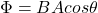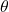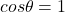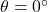a loop of wire is placed in a uniform magnetic field. Let ø be the angle between the direction of b and the normal. At which value of ø is t

Question

a loop of wire is placed in a uniform magnetic field. Let ø be the angle between the direction of b and the normal. At which value of ø is the magnetic flux through the loop greatest?
A. 90 degrees
B. 0 degrees
C. 45 degrees
D. 100 degrees

in progress 0
5 months 2021-08-26T19:40:41+00:00 1 Answers 7 views 0

B. 0 degrees

Explanation:

The magnetic flux through a certain area enclosed by a loop of wire immersed in a region with magnetic field is given by:where

B is the strength of the magnetic field

A is the area enclosed by the coilis the angle between the direction of the magnetic field and the direction of the normal to the coil

From the equation above, we see that the magnetic flux is maximum whenSo when the field is parallel to the normal to the coil (so, when it is perpendicular to the coil), therefore when:So, the correct option is

B. 0 degrees